Imagination is more important than knowledge... Albert Einstein

Guess is more important than calculation --- Knowhowacademy.com

Trigonometry - Trigonometric ratio

For ACT
Number of Total Problems: 35.
FOR PRINT ::: (Book)

 Problem Num : 1 Type: None Section:Trigonometry Theme:None Adjustment# : 0 Difficulty: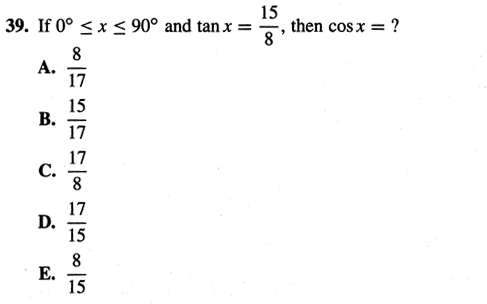Category Trigonometric ratio
Analysis looks like 17 is involved.

 Problem Num : 2 Type: None Section:Trigonometry Theme:None Adjustment# : 0 Difficulty: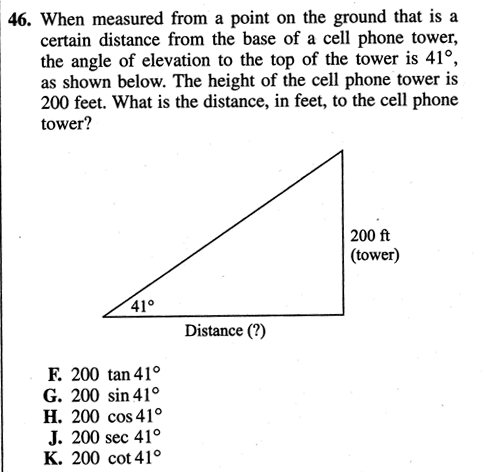Category Trigonometric ratio
Analysis

 Problem Num : 3 Type: None Section:Trigonometry Theme:None Adjustment# : 0 Difficulty: 1Category Trigonometric ratio
Analysis WHAT IS THE MAX OF SIN?

 Problem Num : 4 Type: None Section:Trigonometry Theme:None Adjustment# : 0 Difficulty: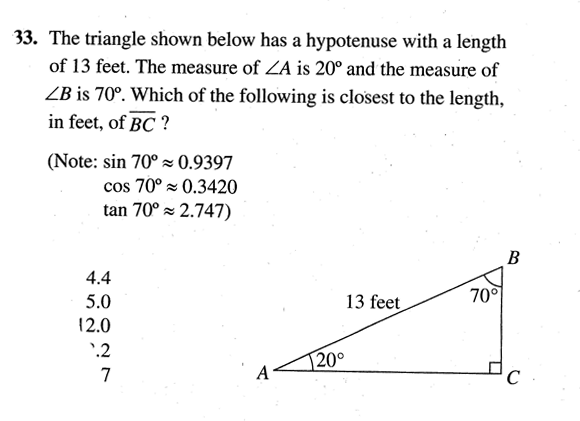Category Trigonometric ratio
Analysis

 Problem Num : 5 Type: None Section:Trigonometry Theme:None Adjustment# : 0 Difficulty: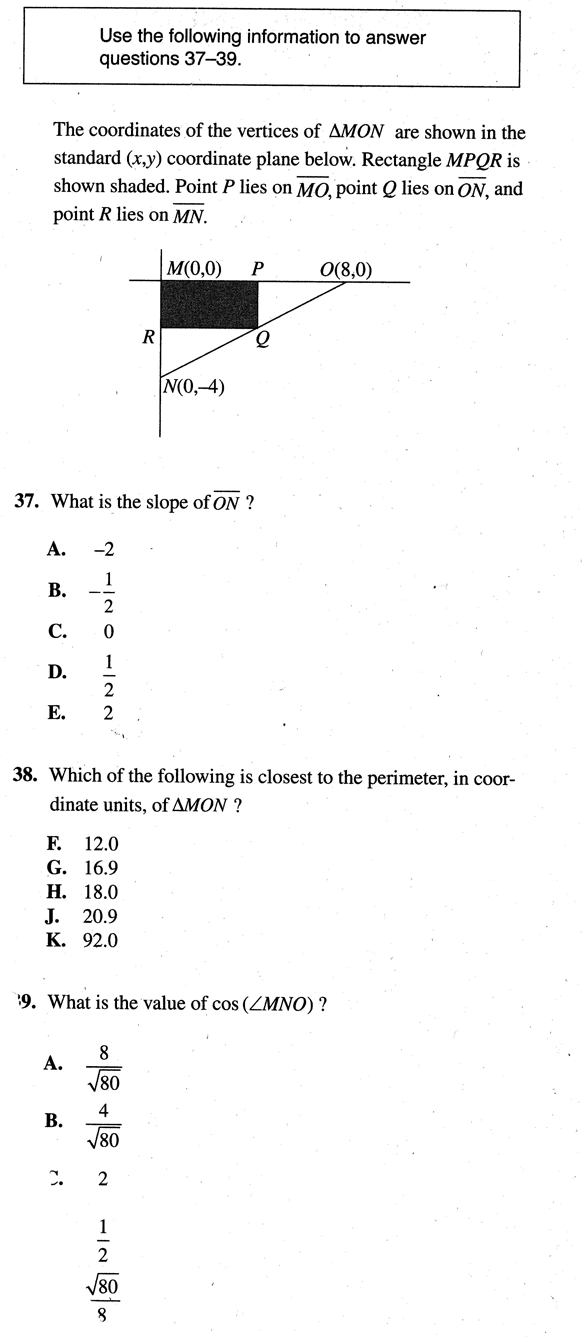Category Trigonometric ratio
Analysis

 Problem Num : 6 Type: None Section:Trigonometry Theme:None Adjustment# : 0 Difficulty: 1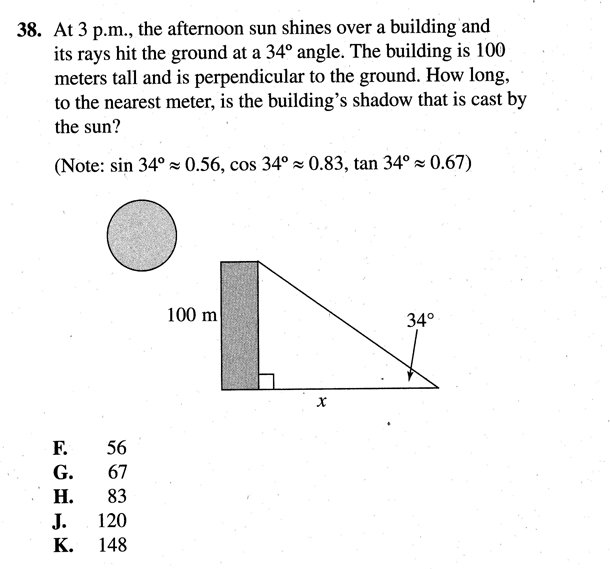Category Trigonometric ratio
Analysis If you divide the number by the decimal (less than 1), what happen?

 Problem Num : 7 Type: None Section:Trigonometry Theme:None Adjustment# : 0 Difficulty: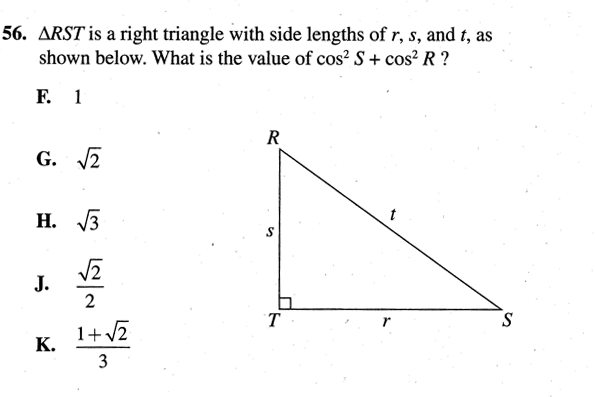Category Trigonometric ratio
Analysis

 Problem Num : 8 Type: None Section:Trigonometry Theme:None Adjustment# : 0 Difficulty: 1Category Trigonometric ratio
Analysis Relationship.

 Problem Num : 9 Type: None Section:Trigonometry Theme:None Adjustment# : 0 Difficulty: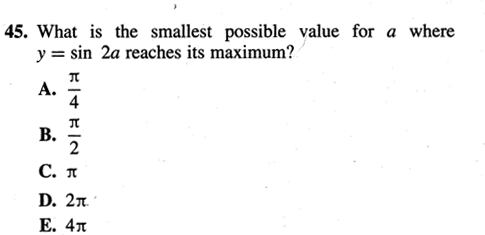Category Trigonometric ratio
Analysis WHAT IS THE MAX OF SIN?

 Problem Num : 10 Type: None Section:Trigonometry Theme:None Adjustment# : 0 Difficulty: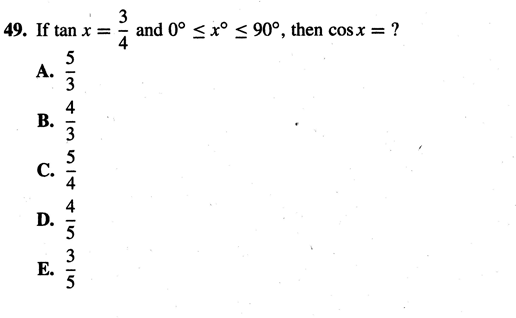Category Trigonometric ratio
Analysis